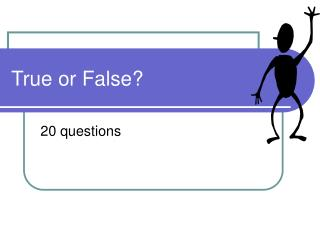DownloadDownload PresentationTrue or False?

# True or False?

Download Presentation## True or False?

- - - - - - - - - - - - - - - - - - - - - - - - - - - E N D - - - - - - - - - - - - - - - - - - - - - - - - - - -
##### Presentation Transcript

1. True or False? 20 questions

2. Question 1 The moment of a force F about a pivot is F/d False - Fxd

3. Question 2 The centre of mass of an object is the point where its mass is spread out. False - concentrated

4. Question 3 A suspended object is in equilibrium when its centre of mass is directly beneath the point of suspension. True

5. Question 4 The centre of mass of a symmetrical object is off the axis of symmetry. False - on

6. Question 5 True - W1d1=W2d2

7. Question 6 The stability of an object is decreased by making its base as wide as possible False - increased

8. Question 7 The stability of an object is increased by making its centre of mass as high as possible. True

9. Question 8 An object will topple over if the line of action of its weight is outside its base. True

10. Question 9 The greater the mass of an object the lower the centripetal force. False - greater

11. Question 10 The Centripetal force increases as the radius of the circle decreases. True

12. Question 11 Gravity is measured in Newtons. False – N/kg

13. Question 12 The force of gravity between two objects is an attractive force. True

14. Question 13 The greater the distance between two objects the greater the force of gravity. False - smaller

15. Question 14 The smaller two objects are the greater the force of gravity. False - smaller

16. Question 15 The larger an orbit is the longer the orbiting body takes to go round. True

17. Question 16 If the speed of a planet in orbit is too high it will spiral into the Sun. False – fly off into space

18. Question 17 If the speed of a planet in orbit is too low it will spiral into the Sun. True

19. Question 18 A Geostationary satellite orbits the earth in 20 hours. False – 24 hours

20. Question 19 Geostationary satellites are used for many purposes Including weather forecasting and police surveillance. False - communications

21. Question 20 Monitoring satellites are in much lower orbits than geostationary. True